# Classical turning points and connection formula

In quantum mechanics, classical turning points and connection formulas are important concepts that help describe the behaviour of wave functions in different regions of a potential energy landscape. These concepts are particularly relevant when studying the quantization of energy levels and the behaviour of particles in potential wells. Let's explore each concept:

1. Classical Turning Points:

• Classical turning points are positions in a one-dimensional potential energy curve where the classical kinetic energy of a particle is zero. At these points, the particle momentarily comes to a halt and changes its direction of motion.

• Mathematically, a classical turning point occurs when the total mechanical energy (sum of kinetic and potential energy) is equal to the potential energy: E = V(x), where E is the total energy, V(x) is the potential energy, and x is the position of the particle.

• Beyond a classical turning point, the kinetic energy becomes negative, and the particle cannot exist there according to classical mechanics.

• In quantum mechanics, classical turning points play a crucial role in understanding the behaviour of wave functions near regions of high potential energy. Wave functions are often attenuated in these regions.

2. Connection Formulas:

• Connection formulas are mathematical expressions that relate the behaviour of a quantum wave function in one region of a potential to its behaviour in another region, particularly near classical turning points.

• These formulas are used to connect the solutions of the Schrödinger equation in different regions of the potential energy curve.

• Near a classical turning point, the potential energy becomes steep, and the wave function's behaviour changes rapidly. The connection formulas help describe how the wave function changes as it transitions from one region to another.

• The most common connection formula is the Airy function, which is used to describe the behaviour of wave functions near classical turning points in one-dimensional potentials.

The Airy function (Ai(x)) is a special function that appears in solutions to the Schrödinger equation in one-dimensional potentials with classical turning points. Its behaviour near the turning point is oscillatory and describes the tunneling of particles through energy barriers.

In summary, classical turning points are positions in a potential energy landscape where the classical kinetic energy is zero, and connection formulas are mathematical tools used to describe the behaviour of quantum wave functions as they transition between regions near classical turning points. These concepts are essential for understanding quantum mechanics in the presence of varying potentials and energy barriers.

The validity of WKB approximation clearly breaks down at the turning points. This happens if k(x) = 0 or it varies rapidly at that points.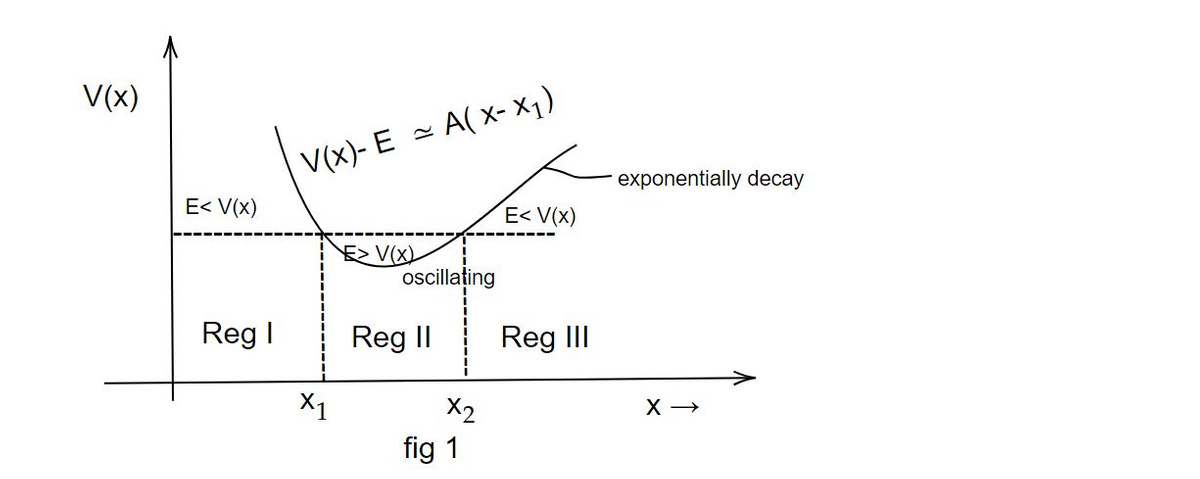i.e V(x) = E ...1

This is the condition of breakdown of WKB approximation.

The potential function in the neighbourhood of x₁ and x₂ are approximated as

V(x) - E ≃ - A (x - x₁) at x = x₁

and V(x) - E ≃ B (x - x₂) at x = x₂ .......2

The Schrodinger equation for those two points are reduced to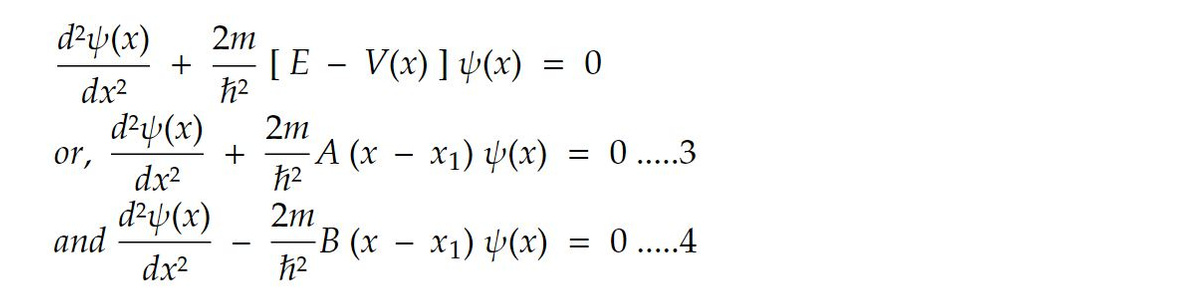Now using the following transformation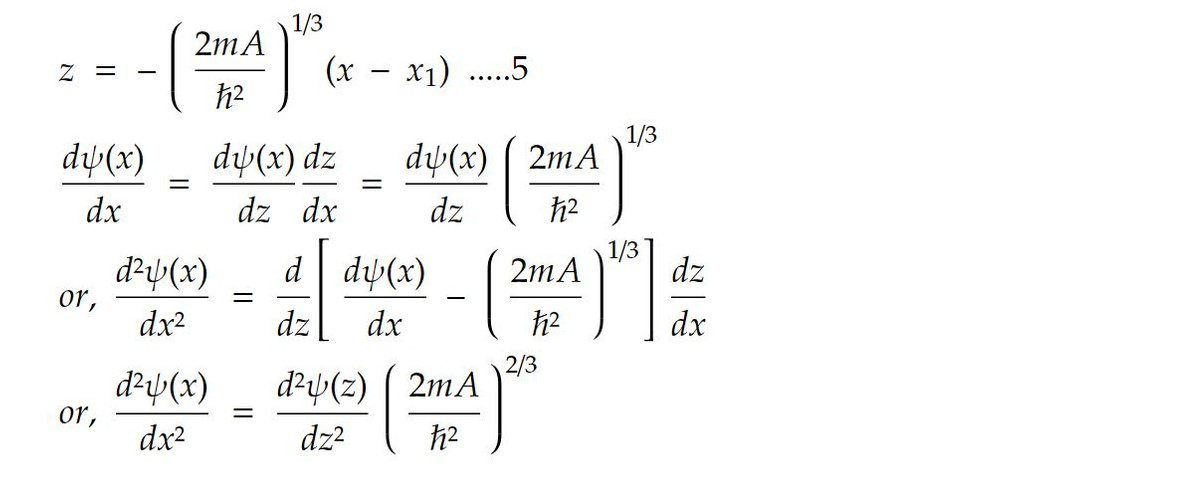Substituting this value in equation 3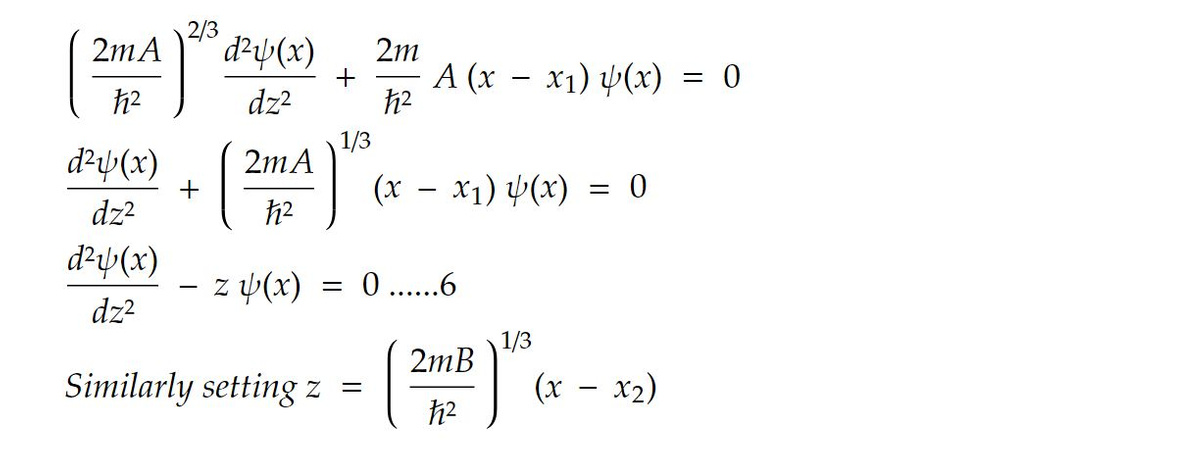in equation 4 we get equation 6 which is called Airy equation.

The solution of Airy function, which should vanish asymptotically for large large of z corresponding to x< x₁ and x> x₁. For real values of z, the Airy function is expressed as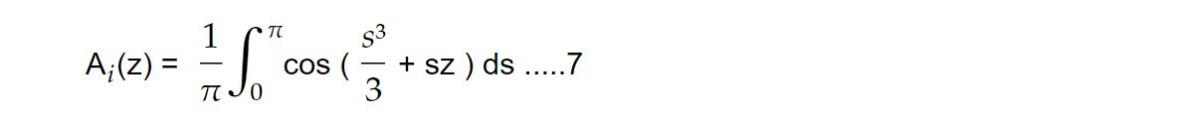Graphically fig 2 represents Airy function.Graphically fig 2 represents Airy function.

This note is a part of the Physics Repository.